Time: 3 Hours                                                                                                     Max. Marks: 100

NOTE: There are 9 Questions in all.

·      Question 1 is compulsory and carries 20 marks. Answer to Q. 1. must be written in the space provided for it in the answer book supplied and nowhere else.

·      Out of the remaining EIGHT Questions answer any FIVE Questions. Each question carries 16 marks.

·      Any required data not explicitly given, may be suitably assumed and stated.

Q.1       Choose the correct or best alternative in the following:                                         (2x10)

a.       The eddy current loss in an a-c electric motor is 100 watts at 50 Hz. Its loss at 100 Hz will be

(A) 25 watts                                        (B) 59 watts

(C) 100 watts                                      (D) 400 watts

b.      The maximum power for a given excitation in a synchronous motor is developed when the power angle is equal to

(A)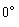(B)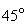(C)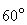(D)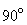c.   A commutator in a d.c. machine

(A)    Reduces power loss in armature.

(B)    Reduces power loss in field circuit.

(C)    converts the induced a-c armature voltage into direct voltage.

(D)    Is not necessary.

d.   The speed of a d.c. shunt motor at no-load is

(A)    5 to 10%                                    (B) 15 to 20%

(C)  25 to 30%                                   (D)  35 to 40%

higher than its speed at rated load.

e.   The efficiency of a transformer is mainly dependent on

(A) core losses.                                  (B) copper losses.

(C)  stray losses.                                 (D) dielectric losses.

f.    When two transformers are operating in parallel, they will share the load as under:

(A)     proportional to their impedances.

(B)     inversely proportional to their impedances.

(C)     50% - 50%

(D)    25%-75%

g.   If the voltage is reduced to half, the torque developed by an induction motor will be reduced to

(A)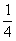of original torque.                    (B)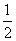of original torque.

(C)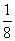of original torque.                     (D)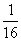of original torque.

h.   A 3-phase, 400 votts, 50 Hz, 100 KW, 4 pole squirrel cage induction motor with a rated slip of 2% will have a rotor speed of

(A)    1500 rpm                                     (B) 1470 rpm

(C) 1530 rpm                                      (D) 1570 rpm

i.    If the phase angle of the voltage coil of a directional relay is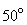, the maximum torque angle of the relay is

(A)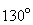(B)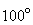(C)(D)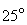j.    The voltage at the two ends of a transmission line are 132 KV and its reactance is 40 ohm. The Capacity of the line is

(A) 435.6 MW                                    (B) 217.8 MW

(C) 251.5 MW                                    (D) 500 MW

Answer any FIVE Questions out of EIGHT Questions.

Each question carries 16 marks.

Q.2           A 50 KVA, 2300/230 V, 60 Hz transformer has a high voltage winding resistance of 0.65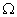and a low-voltage winding resistance of 0.0065. Laboratory tests showed the following results:

Open circuit test:  V = 230 V, I =5.7A, P = 190 W

Short circuit test:  V=41.5 V, I=21.7 A, P=No watt meter was used.

a.   Compute the value of primary voltage needed to give rated secondary voltage when the transformer is connected as a step-up one and is delivening 50 KVA at a power factor of 0.8 lagging.      (12)

b.   Compute the efficiency under conditions of part (a).                                            (4)

Q.3           A three-phase, 335-hp, 2000V, six pole, 60 Hz, Y-connected squirrel-cage induction motor has the following parameters per phase that are applicable at normal slips: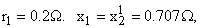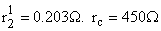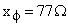The rotational losses are 4100 watts. Using the approximate equivalent circuit, compute for a slip of 1.5%.

(i)                  the line power factor and current.

(ii)                developed torque.

(iii)               efficiency.                                                                          (8+4+4)

Q.4           A 2300-V, three phase, 60 Hz, star-connected cylindrical synchronous motor has a synchronous reactance of 11per phase. When it delivers 200 hp, the efficiency is found to be 90% exclusive of field loss, and the power-angle is 15 electrical degrees as measured by a stroboscope. Neglect ohmic resistance and determine:

(i)                  the induced excitation per phase.

(ii)                the line current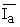(iii)               the power factor                                                                (8+4+4)

Q.5           When a 250-V, 50 hp, 1000 rpm d.c shunt motor is used to supply rated output power to a constant torque load, it draws an armature current of 160A. the armature circuit has a resistance of 0.04and the rotational losses are equal to 2 KW. An external resistance of 0.5is inserted in series with the armature winding. For this condition compute

(i)                  the speed

(ii)                the developed power

(iii)               the efficiency assuming that the field loss is 1.6 K.W                       (8+4+4)

Q.6           Give the lay-out of a typical thermal-power plant and briefly explain the working of the super heater and the condenser.                                           (8+4+4)

Q.7           With a neat diagram describe the construction of  a lead acid cell and explain the process of charging and discharging of the cell.                          (8+4+4)

Q.8     a.   What are the criteria for the classification of transmission lines as short, medium and long lines?                                                                  (8)

b.   Draw the schematic diagram of a directional overcurrent relay and explain its working.                    (4)

c.   What are the advantages of high voltage transmission and its limitations.               (4)

Q.9           Write short  notes on ANY TWO of the following:

(i)                  Capacitor-start motor.

(ii)                Switched reluctance motor.

(iii)               Stepper motor.                                                                      (8+8)